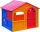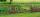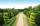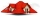# Parcel

Both dimensions of the rectangular parcel were increased by 31%. By how many % has increased its acreage?

x =  71.61 %

### Step-by-step explanation:Did you find an error or inaccuracy? Feel free to write us. Thank you!Tips to related online calculators

#### You need to know the following knowledge to solve this word math problem:

We encourage you to watch this tutorial video on this math problem:

## Related math problems and questions:

• Hop-gardenThe length of the rectangular hop garden Mr. Smith increased by 25% and its width by 30%. What is the percentage change in area of hop garden?
• Percent changeIf the length of a rectangle is increased by 25% and the width is decreased by 10%, the area of the rectangle is larger than the area of the original rectangle by what percent?
• Rectangular landOn a rectangular land with dimensions of 35 m and 18.5 m is a house with a square floor plan with a side of 14 m. What % of the land is not occupied?
• Rectangle areaThe length of a rectangle of x units is increased by 10%, and its width of y units is increased by 15%. What is the ratio of the area of the old rectangle to the area of the new rectangle?
• AcreageWhat acreage has a rectangular plot whose diagonal is 34 meters long and one side has a length of 16 meters. ...
• GardenThe garden has a rectangular shape with lengths of 25 and 40 meters. It has been expanded so that each of its size increased by one fifth. How many square meters increased its acreage?
• PlotThe length of the rectangle is 8, smaller than three times the width. If we increase the width by 5% of the length and the length is reduced by 14% of the width, the circumference of the rectangle will be increased by 30 m. What are the dimensions of the
• Rectangular gardenThe perimeter of Peter's rectangular garden is 98 meters. The width of the garden is 60% shorter than its length. Find the dimensions of the rectangular garden in meters. Find the garden area in square meters.
• RectangleThe width of the rectangle is 65% of its length. Perimeter of the rectangle is 132 cm. Determine the dimensions of the rectangle.
• Rectangular landDue to the new road, the longer dimension of the rectangular plot had to be shortened by 4 percent and the shorter dimension shortened by 10 percent. By how many percent has the area of land decreased?
• Square - increased perimeterHow many times is increased perimeter of the square, where its sides increases by 150%? If the perimeter of square will increase twice, how much% increases the content area of the square?
• Parcelparcel has a rectangular shape of a trapezoid with bases 12 m and 10 m and a height 8 m. On parcel was built object with a footprint an isosceles triangle shape with side 4 m and height three-quarters of a meter. What is the area of unbuild parcel?
• Rectangular plotThe dimensions of a rectangular plot are (x+1)m and (2x-y)m. If the sum of x and y is 3m and the perimeter of the plot is 36m. Find the area of the diagonal of the plot.
• Unknown number 10Number first increased by 30%, then by 1/5. What percentage we've increased the original number?
• TilesThe pupils of the building school have to calculate how many roof tiles they will need to cover the roof of the house in the shape of two rectangles measuring 10 x 7.2 m. One roof tile covers a rectangular area of 22 cmx32 cm. How many tiles will they nee
• Rectangle 35Find the area of a rectangle when the diagonal is equal to 30 cms and the width is double the length.
• Percent calculationCalculate 8% if 44% is 32.